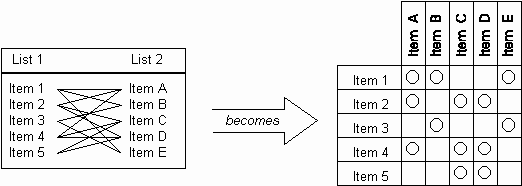The Psychology of Quality and More

# Matrix Diagram

The Matrix Diagram allows a many-to-many comparison of two lists, by turning the second list on its side to form a matrix. The illustration below shows how the relationship between two items can now be indicated in the cell where the row and column of the two items cross.

Common extensions to the Matrix Diagram include the use of different symbols to indicate different comparison levels and the weighting of the items being compared.

There are a number of different shapes of matrix for comparing more than the basic two lists. These include the L-Matrix (the basic matrix as below), C-Matrix, T-Matrix, X-Matrix and Y-Matrix.

The Matrix Diagram is a core tool in Quality Function Deployment (QFD).

The Matrix Diagram is one of the second seven tools.Toolbook chapter: Matrix Diagram

### You can buy books here

And the big
paperback book